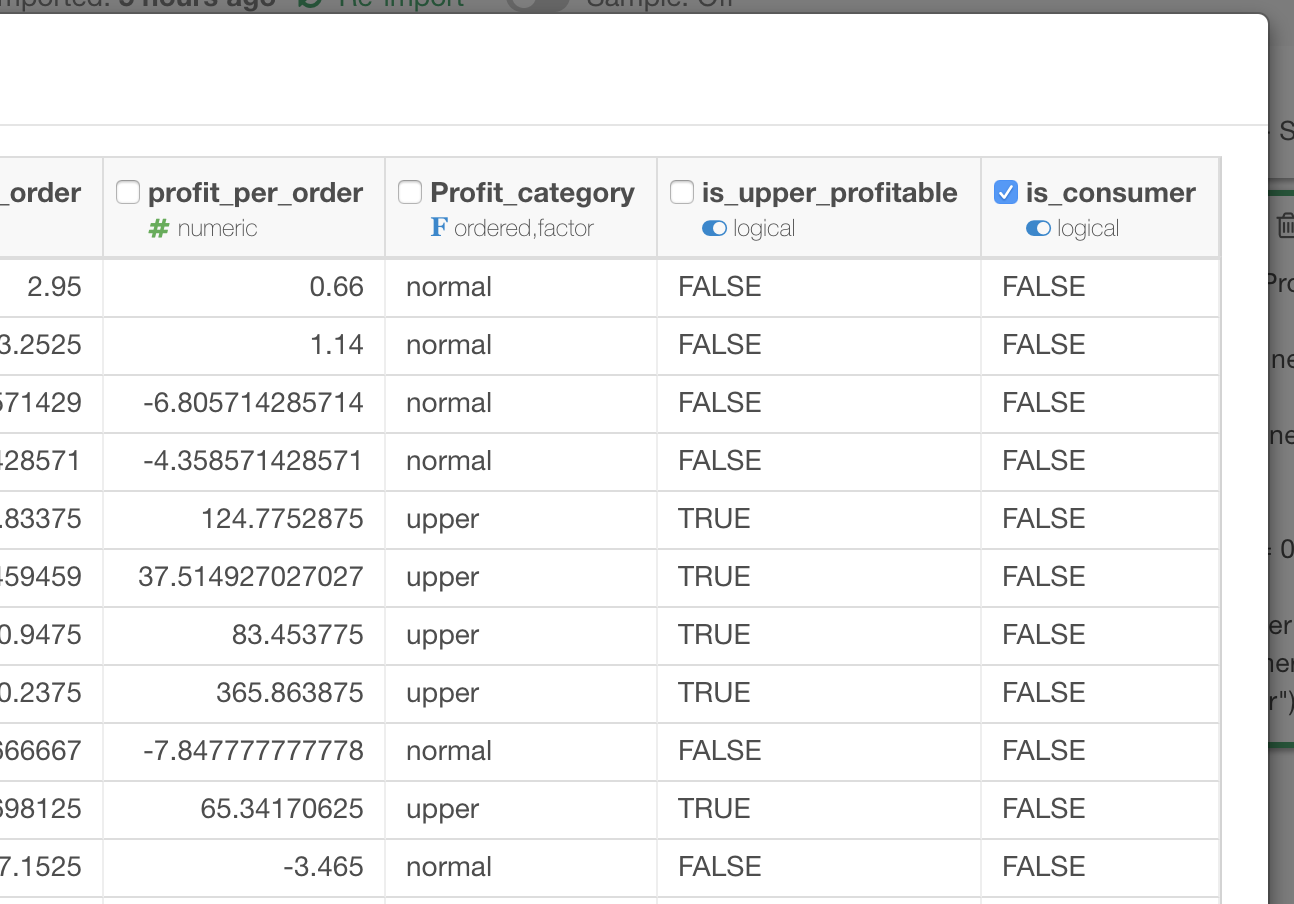# Linear Regression: Some coefficients are not shown on Coefficients view

If you are not seeing some coefficients you specified on Coefficients chart of Linear Regression Analytics View, it might be because of this.
For example, I checked is_consumer column here, as an explanatory variable for linear regression,but I’m not seeing it on Coefficients chart here.

It turned out that is_consumer column is derived from `Customer Segment` column,

and `Customer Segment` column is also included as an explanatory variable of this linear regression.

In this case, R’s linear regression algorithm (lm) figures out that is_consumer being TRUE and `Customer Segment` having the value “Consumer” is exact same thing, and drops one of them from the coefficients, which is is_consumer in this case.